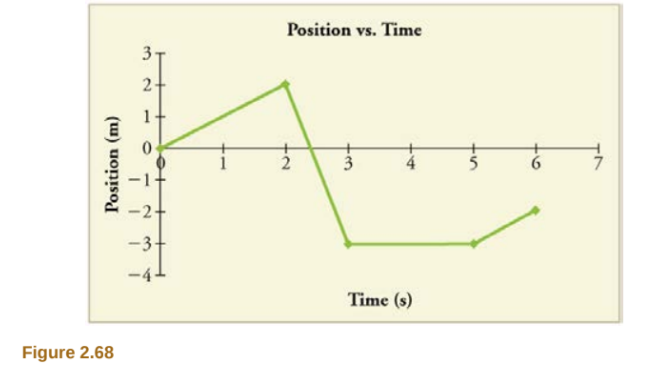×
Get Full Access to College Physics - 1 Edition - Chapter 2 - Problem 66pe
Get Full Access to College Physics - 1 Edition - Chapter 2 - Problem 66pe

×

# Solved: Figure 2.68 shows the displacement graph for aISBN: 9781938168000 42

## Solution for problem 66PE Chapter 2

College Physics | 1st Edition

• Textbook Solutions
• 2901 Step-by-step solutions solved by professors and subject experts
• Get 24/7 help from StudySoup virtual teaching assistantsCollege Physics | 1st Edition

4 5 1 300 Reviews
25
4
Problem 66PE

Figure 2.68 shows the displacement graph for a particle for 5 s. Draw the corresponding velocity and acceleration graphs.Step-by-Step Solution:

SOLUTION TO 66PE

### Step 1 of 2

We need to find the velocity time graph and the acceleration time graph for the body upto 5s during motion. The Position,and time values are given in the table.

 Time Position 0s 0m 2s 2m 3s -3m 5s -3m

### Velocity at a point is the slope of the position time graph at a point. Acceleration is the slope of the velocity time graph at a point.

Using the formulas,is the final positionis the initial positionis the final timeis the initial time.is the final velocityis the initial velocity

Step 2 of 2

##### ISBN: 9781938168000

The answer to “?Figure 2.68 shows the displacement graph for a particle for 5 s. Draw the corresponding velocity and acceleration graphs.” is broken down into a number of easy to follow steps, and 19 words. Since the solution to 66PE from 2 chapter was answered, more than 2335 students have viewed the full step-by-step answer. College Physics was written by and is associated to the ISBN: 9781938168000. This textbook survival guide was created for the textbook: College Physics , edition: 1. The full step-by-step solution to problem: 66PE from chapter: 2 was answered by , our top Physics solution expert on 07/07/17, 04:39PM. This full solution covers the following key subjects: acceleration, corresponding, displacement, draw, figure. This expansive textbook survival guide covers 34 chapters, and 3125 solutions.

Unlock Textbook Solution